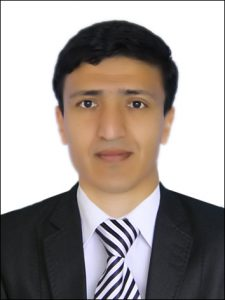# Bulletin KRASEC. Phys. & Math. Sci. 2016. V. 12. no. 1. pp. 5-11. ISSN 2313-0156

Back to contents

DOI: 10.18454/2313-0156-2016-12-1-5-11

MATHEMATICS

MSC 35M10

ON A PROBLEM FOR THE LOADED MIXED TYPE EQUATION WITH FRACTIONAL DERIVATIVE

O.Kh. Abdullayev

National University of Uzbekistan by Mirzo Ulugbeka, 100174, Uzbekistan, Tashkent c., VUZ gorodok st.
E-mail: obidjon.mth@gmail.com

An existence and an uniqueness of solution of local boundary value problem with discontinuous matching condition for the loaded parabolic-hyperbolic equation involving the Caputo fractional derivative and Riemann-Liouville integrals have been investigated in this research work. The uniqueness of solution is proved by the method of integral energy and the existence is proved by the method of integral equations.

Key words: Caputo derivative, boundary value problem, Riemann-Liouville integral, energy integral.

References

1. Kadirkulov B. J.Boundary problems for mixed parabolic-hyperbolic equations with two lines of changing type and fractional derivative. Electronic Journal of Differential Equations. 2014. vol. 2014. no 57. pp. 1–7
2. Karimov E. T., Akhatov J.A boundary problem with integral gluing condition for a parabolichyperbolic equation involving the Caputo fractional derivative. Electronic Journal of Differential Equations. 2014. vol. 2014. no. 14. pp. 1–6
3. Nakhushev A.M.Nagruzhennye uravneniya i ikh prilozheniya [Loaded Equations and Applications]. Moskva. Nauka. 2012. 232 p.
4. Eleev V. A. O nekotorykh kraevykh zadachakh dlya smeshanno-nagruzhennykh uravneniy vtorogo i tret’ego poryadka [Some boundary value problems for mixed-loaded equations of the second and third order]. Differentsial’nye uravneniya — Differential equations. 1994. vol 30. no. 2. pp. 230–236
5. Kaziev V. M. O zadache Darbu dlya odnogo nagruzhennogo integro-differentsial’nogo uravneniya vtorogo poryadka [Darboux problem for a loaded integro-differential equation of second order]. Differentsial’nye uravneniya — Differential equations. 1978. vol 14. no 1. pp. 181–184
6. Lanin I. N. Kraevaya zadacha dlya odnogo nagruzhennogo giperbolo-parabolicheskogo uravneniya tret’ego poryadka [A boundary value problem for a hyperbolic-parabolic loaded third order equations]. Differentsial’nye uravneniya — Differential equations. 1981. vol 17.no 1. pp. 97–106
7. Abdullaev O. Kh. Kraevaya zadacha dlya nagruzhennogo uravneniya elliptiko-giperbolicheskogo tipa v dvusvyaznoy oblasti [A boundary value problem for a loaded equation elliptic-hyperbolic type in a doubly connected region]. Vestnik KRAUNTS. Fiz.-mat. nauki — Bulletin KRASEC. Phys. and Math. Sci. 2014. no 1(8). pp. 33–48
8. Abdullaev O. Kh.About a method of research of the non-local problem for the loaded mixed type equation in double-connected domain. Bulletin KRASEC. Phys. & Math. Sci. 2014. no 9(2). pp. 11–16
9. Abdullaev O. Kh. Non-local boundary value problem for the mixed type equations on the third order in double-connected domains. Journal of Partial Differential Equations. 2014. vol. 27. no 4. pp. 283-292
10. Abdulaev O. Kh.Nelokal’naya zadacha dlya nagruzhennogo uravneniya smeshannogo tipa s integral’nym operatorom. Differentsial’nye uravneniya i matematicheskoe modelirovanie. Mezhdunarodnaya konferentsiya. Ulan-Ude. 2015. pp. 21-23
11. Pskhu A.V. Uravneniye v chasnykh proizvodnykh drobnogo poryadka [Partial differential equations of fractional order]. Moskva. Nauka. 2005. 200 p.
12. Smirnov M. M. Uravneniya smeshannogo tipa [The equations of mixed type]. Moskva. Nauka. 1970. 296 p.
13. Pskhu A.V.Solution of boundary value problems fractional diffusion equation by the Green function method. Differential equation. 2003. vol. 39. no 10. pp. 1509–1513

For citation: Abdullayev O.Kh. On a problem for the loaded mixed type equation with fractional derivative. Bulletin KRASEC. Physical and Mathematical Sciences 2016, vol. 12, no 1, 5-11. DOI: 10.18454/2313-0156-2016-12-1-5-11

Original article submitted: 20.03.2016

1……………………………………………………………………………………

Abdullayev Obidjon Khayrullaevich – Ph.D. (Phys. & Math.), Associate Professor Dept. of Differential equations of the National University of Uzbekistan named after Ulugbek, Uzbekistan.